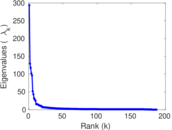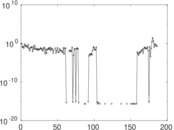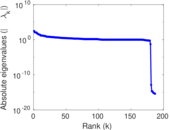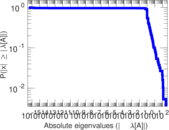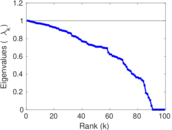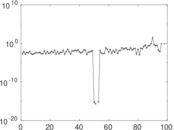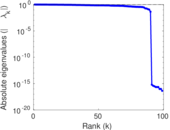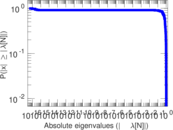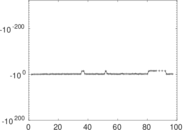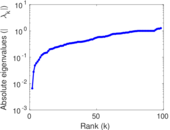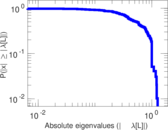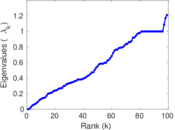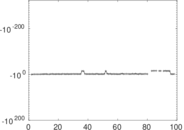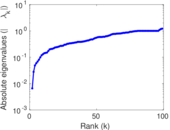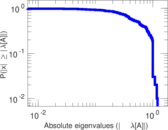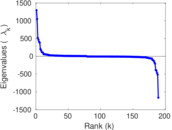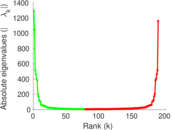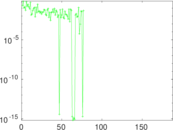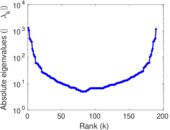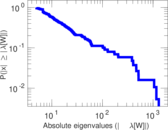# Wikibooks edits (eu)

This is the bipartite edit network of the Basque Wikibooks. It contains users and pages from the Basque Wikibooks, connected by edit events. Each edge represents an edit. The dataset includes the timestamp of each edit.

 Code `beu` Internal name `edit-euwikibooks` Name Wikibooks edits (eu) Data source http://dumps.wikimedia.org/ AvailabilityDataset is available for download Consistency checkDataset passed all tests Category Authorship network Dataset timestamp 2017-10-20 Node meaning User, article Edge meaning Edit Network formatBipartite, undirected Edge typeUnweighted, multiple edges Temporal dataEdges are annotated with timestamps

## Statistics

 Size n = 1,197 Left size n1 = 189 Right size n2 = 1,008 Volume m = 4,898 Unique edge count m̿ = 1,294 Wedge count s = 49,521 Claw count z = 2,301,777 Cross count x = 90,383,767 Square count q = 1,975 4-Tour count T4 = 216,584 Maximum degree dmax = 1,786 Maximum left degree d1max = 1,786 Maximum right degree d2max = 176 Average degree d = 8.183 79 Average left degree d1 = 25.915 3 Average right degree d2 = 4.859 13 Fill p = 0.006 792 22 Average edge multiplicity m̃ = 3.785 16 Size of LCC N = 956 Diameter δ = 13 50-Percentile effective diameter δ0.5 = 4.463 55 90-Percentile effective diameter δ0.9 = 8.037 49 Median distance δM = 5 Mean distance δm = 5.409 22 Gini coefficient G = 0.804 072 Balanced inequality ratio P = 0.167 313 Left balanced inequality ratio P1 = 0.105 553 Right balanced inequality ratio P2 = 0.222 131 Relative edge distribution entropy Her = 0.840 057 Power law exponent γ = 4.749 14 Tail power law exponent γt = 2.571 00 Tail power law exponent with p γ3 = 2.571 00 p-value p = 0.000 00 Left tail power law exponent with p γ3,1 = 1.811 00 Left p-value p1 = 0.752 000 Right tail power law exponent with p γ3,2 = 2.971 00 Right p-value p2 = 0.000 00 Degree assortativity ρ = −0.177 514 Degree assortativity p-value pρ = 1.271 60 × 10−10 Spectral norm α = 293.729 Algebraic connectivity a = 0.006 555 60 Spectral separation |λ1[A] / λ2[A]| = 2.267 29 Controllability C = 824 Relative controllability Cr = 0.695 946

## Plots

### Fruchterman–Reingold graph drawing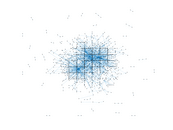### Degree distribution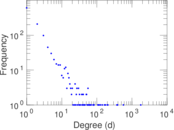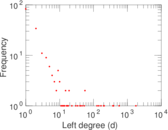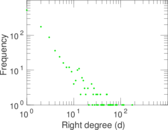### Cumulative degree distribution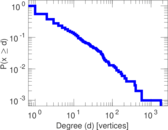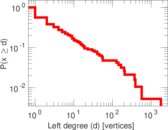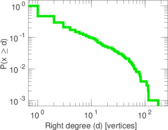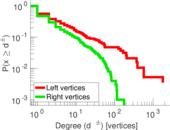### Lorenz curve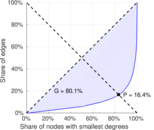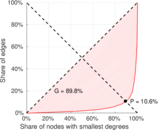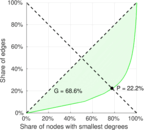### Spectral distribution of the adjacency matrix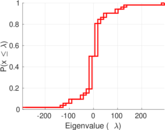### Spectral distribution of the normalized adjacency matrix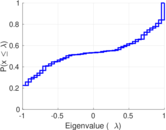### Spectral distribution of the Laplacian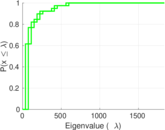### Spectral graph drawing based on the adjacency matrix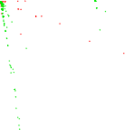### Spectral graph drawing based on the Laplacian### Spectral graph drawing based on the normalized adjacency matrix### Degree assortativity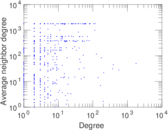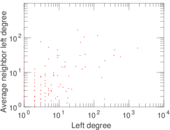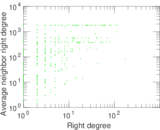### Zipf plot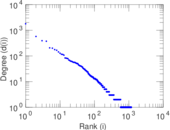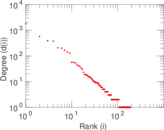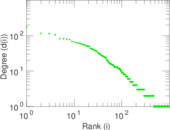### Hop distribution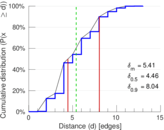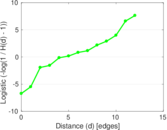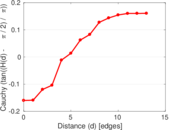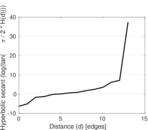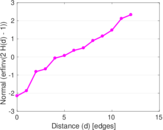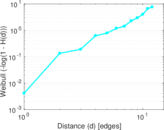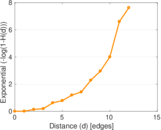### Double Laplacian graph drawing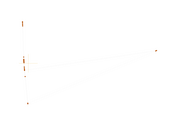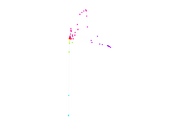### Delaunay graph drawing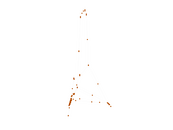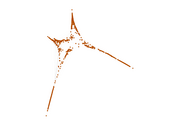### Edge weight/multiplicity distribution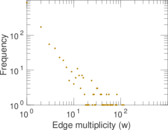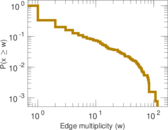### Temporal distribution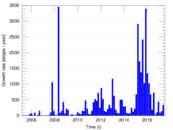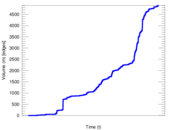### Temporal hop distribution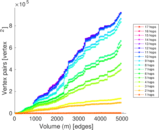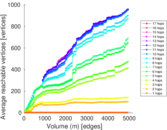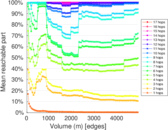### Diameter/density evolution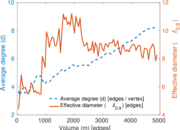### Matrix decompositions plots